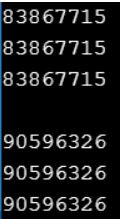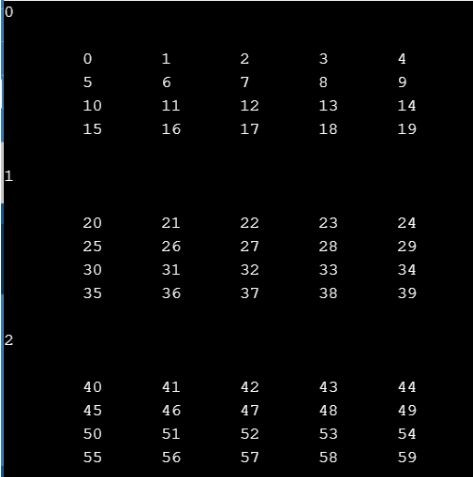# Dynamic Array Creation in C

## Dynamic Array Creation in C Language with Examples

In this article, I am going to discuss Dynamic Array Creation in C Programming Language with Examples. Please read our previous articles, where we discussed Dynamic Memory Management in C. At the end of this article, you will understand what are dynamic arrays, why we need a dynamic array, and how to create a dynamic array with Examples.

##### Dynamic Array:

Static array variables are fixed in size. They are linked in the data area or stack area or in the const area depending on the declaration. Now, this element group is fixed and cannot be changed. To affect this shortcoming dynamic arrays are often defined. Dynamic array is nothing but allocated during run time with malloc/calloc.

##### Dynamic 2D Array Creation in C Language

There are the following ways to dynamically allocate a 2D array in C Language:

##### Single Pointer

In this method, we simply allocate memory of size M*N dynamically and assign it to the pointer. Even though the memory is linearly allocated, we can use pointer arithmetic to index the 2D array.

##### Program for 2D Array Creation using Single Pointer in C
```#include <stdio.h>
#include <stdlib.h>
int main ()
{
int r = 3, c = 4;
int *arr = (int *) malloc (r * c * sizeof (int));

int i, j, count = 0;
for (i = 0; i < r; i++)
for (j = 0; j < c; j++)
*(arr + i * c + j) = ++count;

for (i = 0; i < r; i++)
for (j = 0; j < c; j++)
printf ("%d ", *(arr + i * c + j));

return 0;
}
```

Output: 1 2 3 4 5 6 7 8 9 10 11 12

##### Pointer to a Pointer (Double Pointer)

To develop a 2D array dynamically, we are required to take a pointer to the pointer variable then one array is required to create and manage multiple rows.

##### Program for 2D Array Dynamic Creation using Pointer to Pointer in C
```#include <stdio.h>
#include <stdlib.h>
int main ()
{
int r = 3, c = 4, i, j, count;
int **arr = (int **) malloc (r * sizeof (int *));

for (i = 0; i < r; i++)
arr[i] = (int *) malloc (c * sizeof (int));

count = 0;
for (i = 0; i < r; i++)
for (j = 0; j < c; j++)
arr[i][j] = ++count;

for (i = 0; i < r; i++)
for (j = 0; j < c; j++)
printf ("%d ", arr[i][j]);

return 0;
}
```

Output: 1 2 3 4 5 6 7 8 9 10 11 12

##### Dynamic 3D Array Creation in C Language

There are the following ways to dynamically allocate a 3D array in C language:

##### Single Pointer

In this approach, we simply allocate memory of size M*N*O dynamically and assign it to a pointer. Even though the memory is linearly allocated, we can use pointer arithmetic to index the 3D array.

##### Program for 3D Array Dynamic Creation using Single Pointer in C
```#include<stdio.h>
#include<stdlib.h>
#define M 2
#define N 3
#define O 4
int main ()
{
int i, j, k;
int *A = (int *) malloc (M * N * O * sizeof (int));
if (A == NULL)
{
fprintf (stderr, "Out of Memory");
exit (0);
}
for (i = 0; i < M; i++)
for (j = 0; j < N; j++)
for (k = 0; k < O; k++)
*(A + i * N * O + j * O + k) = rand () % 100;

for (i = 0; i < M; i++)
{
for (j = 0; j < N; j++)
{
for (k = 0; k < O; k++)
printf ("%d", *(A + i * N * O + k));
printf ("\n");
}
printf ("\n");
}
free (A);
return 0;
}
```
###### Output:##### Triple Pointer:

Implementing multi-dimensional arrays in C is very similar, we use malloc()\free() stdlib methods instead of the new\delete keywords.

##### Program for 3D Array Creation using Triple Pointer in C Language
```#include <stdio.h>
#include <stdlib.h>
void main ()
{
int x = 3, y = 4, z = 5;
int i, j, k;
int *allElements = malloc (x * y * z * sizeof (int));
int ***array3D = malloc (x * sizeof (int **));

for (i = 0; i < x; i++)
{
array3D[i] = malloc (y * sizeof (int *));
for (j = 0; j < y; j++)
{
array3D[i][j] = allElements + (i * y * z) + (j * z);
}
}

for (i = 0; i < x; i++)
{
printf ("%d\n", i);
for (j = 0; j < y; j++)
{
printf ("\n");
for (k = 0; k < z; k++)
{
array3D[i][j][k] = (i * y * z) + (j * z) + k;
printf ("\t%d", array3D[i][j][k]);
}
}
printf ("\n\n");
}

free (allElements);
for (i = 0; i < x; i++)
{
free (array3D[i]);
}
free (array3D);
}
```
###### Output: